<![CDATA[Math Is Fun Forum / Formulas]]> 2021-12-08T08:51:45Z FluxBB https://www.mathisfunforum.com/index.php <![CDATA[Euler's polyhedron formula]]>Euler's polyhedron formula

Let's begin by introducing the protagonist of this story - Euler's formula:

V - E + F = 2.

Simple though it may look, this little formula encapsulates a fundamental property of those three-dimensional solids we call polyhedra, which have fascinated mathematicians for over 4000 years. Actually I can go further and say that Euler's formula tells us something very deep about shape and space. The formula bears the name of the famous Swiss mathematician Leonhard Euler (1707 - 1783), who would have celebrated his 300th birthday this year.

What is a polyhedron?

Before we examine what Euler's formula tells us, let's look at polyhedra in a bit more detail. A polyhedron is a solid object whose surface is made up of a number of flat faces which themselves are bordered by straight lines. Each face is in fact a polygon, a closed shape in the flat 2-dimensional plane made up of points joined by straight lines.

A polygon is called regular if all of its sides are the same length, and all the angles between them are the same; the triangle and square in figure 1 and the pentagon in figure 2 are regular.

A polyhedron is what you get when you move one dimension up. It is a closed, solid object whose surface is made up of a number of polygonal faces. We call the sides of these faces edges - two faces meet along each one of these edges. We call the corners of the faces vertices, so that any vertex lies on at least three different faces. To illustrate this, here are two examples of well-known polyhedra.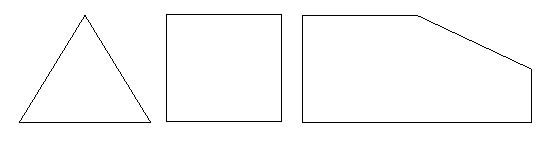A polyhedron consists of just one piece. It cannot, for example, be made up of two (or more) basically separate parts joined by only an edge or a vertex. This means that neither of the following objects is a true polyhedron.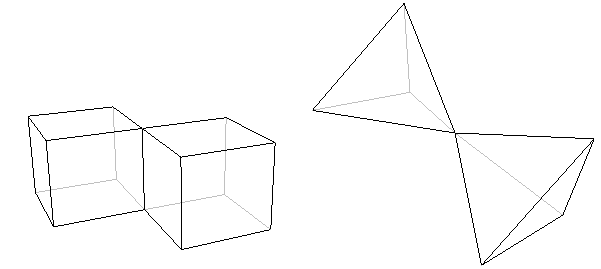What does the formula tell us?
We're now ready to see what Euler's formula tells us about polyhedra. Look at a polyhedron, for example the cube or the icosahedron above, count the number of vertices it has, and call this number V. The cube, for example, has 8 vertices, so V = 8. Next, count the number of edges the polyhedron has, and call this number E. The cube has 12 edges, so in the case of the cube E = 12. Finally, count the number of faces and call it F. In the case of the cube, F = 6. Now Euler's formula tells us that

V - E + F = 2;
or, in words: the number of vertices, minus the number of edges, plus the number of faces, is equal to two.

In the case of the cube, we've already seen that V = 8, E = 12 and F = 6. So,

V - E + F = 8 - 12 + 6 = 14 - 12 = 2
which is what Euler's formula tells us it should be. If we now look at the icosahedron, we find that V = 12, E = 30 and F = 20. Now,

V - E + F = 12 - 30 + 20 = 32 - 30 = 2,
as we expected.
Euler's formula is true for the cube and the icosahedron. It turns out, rather beautifully, that it is true for pretty much every polyhedron. The only polyhedra for which it doesn't work are those that have holes running through them like the one shown in the figure below.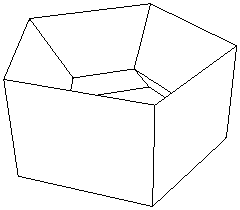These polyhedra are called non-simple, in contrast to the ones that don't have holes, which are called simple. Non-simple polyhedra might not be the first to spring to mind, but there are many of them out there, and we can't get away from the fact that Euler's Formula doesn't work for any of them. However, even this awkward fact has become part of a whole new theory about space and shape.

The power of Euler's formula

Whenever mathematicians hit on an invariant feature, a property that is true for a whole class of objects, they know that they're onto something good. They use it to investigate what properties an individual object can have and to identify properties that all of them must have. Euler's formula can tell us, for example, that there is no simple polyhedron with exactly seven edges. You don't have to sit down with cardboard, scissors and glue to find this out - the formula is all you need. The argument showing that there is no seven-edged polyhedron is quite simple, so have a look at it if you're interested.

Using Euler's formula in a similar way we can discover that there is no simple polyhedron with ten faces and seventeen vertices. The prism shown below, which has an octagon as its base, does have ten faces, but the number of vertices here is sixteen. The pyramid, which has a 9-sided base, also has ten faces, but has ten vertices. But Euler's formula tells us that no simple polyhedron has exactly ten faces and seventeen vertices.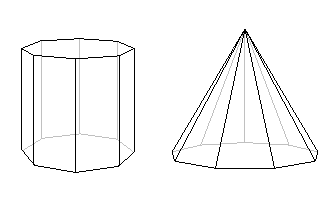It's considerations like these that lead us to what's probably the most beautiful discovery of all. It involves the Platonic Solids, a well-known class of polyhedra named after the ancient Greek philosopher Plato, in whose writings they first appeared.Although their symmetric elegance is immediately apparent when you look at the examples above, it's not actually that easy to pin it down in words. It turns out that it is described by two features. The first is that Platonic solids have no spikes or dips in them, so their shape is nice and rounded. In other words, this means that whenever you choose two points in a Platonic solid and draw a straight line between them, this piece of straight line will be completely contained within the solid — a Platonic solid is what is called convex. The second feature, called regularity, is that all the solid's faces are regular polygons with exactly the same number of sides, and that the same number of edges come out of each vertex of the solid.

The cube is regular, since all its faces are squares and exactly three edges come out of each vertex. You can verify for yourself that the tetrahedron, the octahedron, the icosahedron and the dodecahedron are also regular.

Now, you might wonder how many different Platonic Solids there are. Ever since the discovery of the cube and tetrahedron, mathematicians were so attracted by the elegance and symmetry of the Platonic Solids that they searched for more, and attempted to list all of them. This is where Euler's formula comes in. You can use it to find all the possibilities for the numbers of faces, edges and vertices of a regular polyhedron.What you will discover is that there are in fact only five different regular convex polyhedra! This is very surprising; after all, there is no limit to the number of different regular polygons, so why should we expect a limit here? The five Platonic Solids are the tetrahedron, the cube, the octahedron, the icosahedron and the dodecahedron shown above.

]]>
https://www.mathisfunforum.com/profile.php?id=682 2021-12-08T08:51:45Z https://www.mathisfunforum.com/viewtopic.php?id=26849&action=new
<![CDATA[Formula for Pi using the Nilakantha Series]]> To find a particular term in the series, assuming that the term number is x, replace ∞ with x-1, which works for everything except the first term which is of course 3.

]]>
https://www.mathisfunforum.com/profile.php?id=222180 2021-10-02T19:57:46Z https://www.mathisfunforum.com/viewtopic.php?id=26663&action=new
<![CDATA[Cannot find formula or solution to get answer for feet/inch addition]]> Hi Nickm62388;

Here's my way of tackling these (others may have different methods).

Problem #1
The inches & fraction components in the first length are less than their counterparts in the second length, which adds difficulty to subtracting imperial lengths, particularly those with fractions.

To make the subtraction easier, I start with the following three steps:
1. convert the fraction to the Least Common Denominator (LCD); then
2. adjust the value of the fraction component to make it greater than that of the second length; then
3. adjust the value of the inches component to make it greater than that of the second length.

That leaves a simple subtraction exercise.

Btw, the 'take' and 'add' terminology that I used is like 'borrow' and 'pay', now aka 'regrouping', and is how I've always understood that concept.

Also, I've used symbols ' and " instead of abbreviations 'ft' and 'in' respectively...a common notation practice for these imperial length units.Formula: You could convert both lengths to eighths of an inch, deduct the second from the first, and convert the result to feet, inches and eighths:

That last conversion from eighths is a bit tricky...

Problem #2
Same approach as for Problem #1:Formula: You could convert both angles to seconds, deduct the second from the first, and convert the result to degrees, minutes & seconds:

]]>
https://www.mathisfunforum.com/profile.php?id=40741 2021-07-01T05:29:19Z https://www.mathisfunforum.com/viewtopic.php?id=26416&action=new
<![CDATA[I am confused]]> None of these

]]>
https://www.mathisfunforum.com/profile.php?id=236935 2021-06-02T14:22:47Z https://www.mathisfunforum.com/viewtopic.php?id=24712&action=new
<![CDATA[common mistake]]> Hostible wrote:

Know that -a^2 means -1 x a^2 and not -a x -a.
The reason is that -a^2 has its coefficient as -1
Therefore -a x -a = a^2 and and not -a^2.
This symbol ^ implies exponent.

True but (-a)^2 is totally different.

We know that (-a)^2 = (-a)(-a) = a^2.

Let me see.

-[(a)(a)]

-(a^2)

-a^2

Agree?

]]>
https://www.mathisfunforum.com/profile.php?id=223890 2021-05-15T16:50:47Z https://www.mathisfunforum.com/viewtopic.php?id=19547&action=new
<![CDATA[Elegant Cosine, Heron's and Half Angle Formulae using semiperimeter]]> The Cosine Rule with its squares of sides a , b and c cries out for a fusion with the semiperimeter.

First lets turn the semiperimeter into a few substitutions for later use

Where semiperimeter = s  = ( a + b + c ) / 2

( a + b + c )  = 2 s
( a + b -  c )   =2 ( s – c )
( a -  b + c )   =2 ( s – b )
( b + c -  a )   =2 ( s – a )

Then our Cosine rule

a² = b² +  c² – 2bcCos A

Cos A = ( b² +  c² -  a² ) / 2bc

1 – Cos A = ( 2bc - b² -  c² +  a² ) / 2bc
= ( a – b + c )( a + b - c ) / 2bc
= 2 ( s - b )( s – c ) /  bc

1 + Cos A = ( 2bc + b² +  c² -  a² ) / 2bc
= ( a + b + c )( b + c - a ) / 2bc
= 2 s ( s – a ) /  bc

Sin² A = ( 1 – Cos a )( 1 + Cos A )
= 4 s ( s – a )( s – b )( s - c ) / b²c²

_______________________
Sin A = 2 ( √  s ( s – a )( s – b )( s - c )  ) / bc

Area of Triangle =  ½ . bc Sin A
______________________
=  √  s ( s – a )( s – b )( s - c )   Heron's Area Formula

A much more elegant way of deriving Heron's Formula and there is still the Half angle Formula to go :-

______________
Cos ( A / 2 ) =  √  ( 1 + Cos A ) / 2
______________
=  √  s  (  s – a ) / bc

______________
Sin ( A / 2 ) =  √  ( 1 - Cos A ) / 2
___________________
=  √  ( s – b )(  s – a ) / bc

& Sin A  = 2 Sin ( A / 2 ) Cos ( A / 2 ) gives the same Sin A result as above

Tan ( A / 2 ) = Sin ( A / 2 ) / Cos ( A / 2 )
_______________________
=  √ ( s – b )( s – c ) / s ( s – a )

corrected mistake in half angle formulae

]]>
https://www.mathisfunforum.com/profile.php?id=223802 2021-03-23T14:27:04Z https://www.mathisfunforum.com/viewtopic.php?id=26051&action=new
<![CDATA[Modern Algebra Symbols]]> You have probably solved the problem by now.

Its the geometric symbol for congruent too , and not in open office function editor, which calls the equivalance symbol congruent .

The best stop gap solution would be to screen shot your formula and edit in a photoshop type editor.

I use gimp which is a free editor and just as powerful .

If you then search Google images for ' congruent symbol ' you can copy the one you prefer to your clipboard and paste it in.

There are a lot of formula editors on the market all have limitations but you are using the best of the bunch I think.

]]>
https://www.mathisfunforum.com/profile.php?id=223802 2021-03-20T21:42:23Z https://www.mathisfunforum.com/viewtopic.php?id=24434&action=new
<![CDATA[Primality test and easy factorization]]> Hugo28036 wrote:

This is an algorithm that test if one number is prime and if the number it´s not prime returns the biggest factor of that number.

Are you going to keep it a secret and profit off of it or are you going to enlighten our poor souls as to what it is?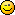]]>
https://www.mathisfunforum.com/profile.php?id=188456 2020-09-11T23:46:17Z https://www.mathisfunforum.com/viewtopic.php?id=25864&action=new
<![CDATA[Proof of the Twin primes Conjecture and Goldbach's conjecture]]> Proof of the Twin primes Conjecture and Goldbach's conjecture
We can find infinite prime numbers with the separation we want and we can express every even number as the sum of two prime numbers.
Proof of the Twin primes Conjecture and Goldbach's conjecture

]]>
https://www.mathisfunforum.com/profile.php?id=222504 2020-08-15T06:13:34Z https://www.mathisfunforum.com/viewtopic.php?id=25831&action=new
<![CDATA[Sum of first n natural numbers - fourth, fifth, and sixth powers.]]> Hi,

.

We know

Sum of first n natural numbers is

Sum of squares of first n natural numbers is

Sum of cubes of first n natural numbers is

In a similar manner, this is additional information.

Sum of fourth powers of n natural numbers is

Sum of fifth powers of n natural numbers is

Sum of sixth powers of n natural numbers is

]]>
https://www.mathisfunforum.com/profile.php?id=682 2020-07-16T05:00:41Z https://www.mathisfunforum.com/viewtopic.php?id=25783&action=new
<![CDATA[Do mental multiplication quickly]]> [deleted, please post if you need this]

]]>
https://www.mathisfunforum.com/profile.php?id=222180 2020-06-29T18:04:47Z https://www.mathisfunforum.com/viewtopic.php?id=25746&action=new
<![CDATA[Decimal to Fraction]]> Hi,

Since edited.

]]>
https://www.mathisfunforum.com/profile.php?id=682 2019-09-06T03:12:10Z https://www.mathisfunforum.com/viewtopic.php?id=25099&action=new
<![CDATA[Solid Geometry Formulas]]> irspow wrote:

or...

A = s²/(4tan[180/n]) where s is length of side and n is number of sides (for those who don't want to use pi to calculate the area of a polygon)Unfortunately, trigonometry tends to turn more people off than π.

]]>
https://www.mathisfunforum.com/profile.php?id=212031 2019-04-18T08:08:29Z https://www.mathisfunforum.com/viewtopic.php?id=3292&action=new
<![CDATA[Tests For Divisibility]]> Here’s a YouTube video on tests of divisibility and how to apply them to some math problems.

[list=*]
[/list]

I found the solution to finding all palindromic four-digit powers particularly interesting.

]]>
https://www.mathisfunforum.com/profile.php?id=217821 2018-07-24T22:21:40Z https://www.mathisfunforum.com/viewtopic.php?id=13168&action=new
<![CDATA[Trigonometry Formulas]]> ganesh wrote:

(Angles are given in degrees, 90 degrees, 180 degrees etc.)

I.
Sin(-θ)=-Sinθ
Cos(-θ) = Cosθ
tan(-θ) = -tanθ
cot(-θ) = -cotθ
sec(-θ) = secθ
cosec(-θ)= - cosecθ

II.
sin(90-θ) = cosθ
cos(90-θ) = sinθ
tan(90-θ) = cotθ
cot(90-θ) = tanθ
sec(90-θ) = cosecθ
cosec(90-θ) = secθ

III.
sin(90+θ) = cosθ
cos(90+θ) = -sinθ
tan(90+θ) = -cotθ
cot(90+θ) = -tanθ
sec(90+θ) = -cosecθ
cosec(90+θ) = secθ

IV.
sin(180-θ) = sinθ
cos(180-θ) = -cosθ
tan(180-θ) = -tanθ
cot(180-θ) = cotθ
sec(180-θ) = -secθ
cosec(180-θ) = cosecθ

V.
sin(180+θ) = -sinθ
cos(180+θ) = -cosθ
tan(180+θ) = tanθ
cot(180+θ) = cotθ
sec(180+θ) = -secθ
cosec(180+θ) = -cosecθ

cot(180-θ)=-cotθ
since cot(180-θ)=1/tan(180-θ)

]]>
https://www.mathisfunforum.com/profile.php?id=219080 2018-04-27T08:48:10Z https://www.mathisfunforum.com/viewtopic.php?id=3290&action=new